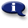# .

## Syllabus Information

Winter 2022
May 18,2022Use this page to maintain syllabus information, learning objectives, required materials, and technical requirements for the course.

Syllabus Information
MTH 243 - Introduction to Probability and Statistics
Associated Term: Winter 2022
Learning Objectives: Upon successful completion of this course, the student should be able to: 1. Classify data as categorical or quantitative. 2. Construct frequency bar charts, histograms, boxplots, stemplots and scatterplots. 3. Use descriptive statistics for center, location and spread. 4. Demonstrate an understanding of probability experiments, outcomes and events. 5. Recognize disjoint and independent events. 6. Find and interpret theoretical and statistical probabilities, including compound and conditional probabilities. 7. Find probabilities and values for a normal distribution. 8. Find probabilities for uniform, binomial, and Poisson distributions 9. Use the Central Limit Theorem to construct confidence intervals for population mean. 10. Construct confidence intervals for population proportion. 11. Test hypotheses about one or two population means. 12. Test hypotheses about one or two population proportions. 13. Interpret the correlation coefficient and its square. 14. Create regression lines and test their statistical significance. 15. Test the independence of two categorical variables using Chi-square. 16. Demonstrate an understanding of sampling and basic research design. 17. Demonstrate an understanding of sources of bias and error in statistical research. 18. Use statistical software and the statistical features of a graphing calculator.
Required Materials:
Technical Requirements: